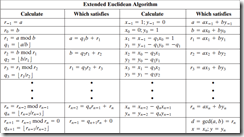## Sunday, June 10, 2012

### Extended GCD algorithm (Extended Euclidean Algorithm)

I had exercise to implement the extended GCD

The aim of this algorithm is to find x,y that satisfies ax + by = gcd(a,b) for any number a,b

The algorithm is as per the text book as below`        public static BigInteger[] Extended_GCD(BigInteger A, BigInteger B)        {            BigInteger[] result = new BigInteger;            if (A < B) //if A less than B, switch them            {                 BigInteger temp = A;                A = B;                B = temp;            }            BigInteger r = B;             BigInteger q = 0;             BigInteger x0 = 1;            BigInteger y0 = 0;            BigInteger x1 = 0;            BigInteger y1 = 1;            BigInteger x = 0, y = 0;            while (r > 1)            {                r = A % B;                q = A / B;                x = x0 - q * x1;                y = y0 - q * y1;                x0 = x1;                y0 = y1;                x1 = x;                y1 = y;                A = B;                B = r;            }            result = r;            result = x;            result = y;            return result;        }`